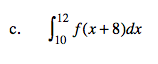### Home > CALC > Chapter 4 > Lesson 4.2.3 > Problem4-77

4-77.
1. If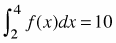find: Homework Help ✎

1.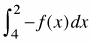2.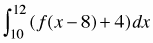3.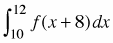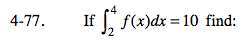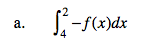Notice that the bounds have flipped and the integrand is now negative.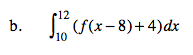Split this problem into a sum of two integrals:

$\int_{10}^{12}f(x-8)dx+\int_{10}^{12}4dx$

Integral 1: Notice that the bounds shifted 8 units to the right... but so did the integrand: f(x − 8).

$\int_{10}^{12}f(x-8)dx=\int_{2}^{4}f(x)dx=10.$

Integral 2: This integral represents a rectangular area with base: 12 − 10 = 2 and height: 4.

$\int_{10}^{12}4dx=(2)(4)=8$